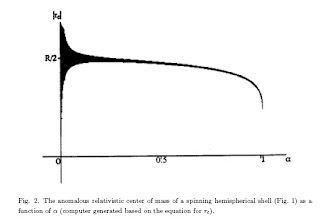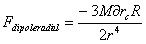## Monday, April 30, 2007

### Radial Component of Dipole Gravity Force in the Equatorial Plane

To calculate the length of the jets from the rotating sphere solely from the linearized theory, one can simply use the following potential.To find the distance r that makes the above total potential zero on the domed side of the rotation axis using the fact that the maximum length allowed for the relativistic shift of the center of mass is R/2 where R is the radius of the sphere(see below diagram), the distance r that defines the length of the jets becomes barely an order of the radius of the sphere R.http://www.tachyonics.com/9604044.pdf .

This is not enough to account for the observed length of the jets. There are many factors that may affect this outcome. The actual geometrical shape of the rotating center could be one of the main factors. If the galactic center is no longer shaped like a sphere but widely stretched disk shape in its solid structure, it will certainly affect the length of the jets in such a way that it extends further away from the center.

There could also be a significant contribution from the non linear effect that the linearized theory could not have taken it into account because the system will no longer be in the weak field regime.

At least for the time being, for the purpose of simulation as a cosmological model , one may have to use the dipole moment (mass times the center of mass shift) as a parameter that determines the strength of the jets. This may eventually help determine the actual geometrical shape of the galactic center.The radial component of the gravito magnetic force around the equatorial plane in the above has M delta Rc dependency which is the major parameter in determining the length of the jets. Here, we can see that the experimental correlations can be made between the length of the jets and the parameters that determine the velocity curve in the dark matter problems of cosmology.